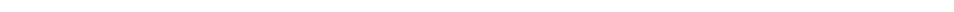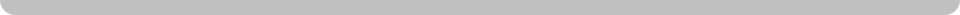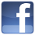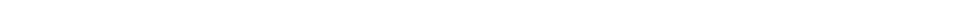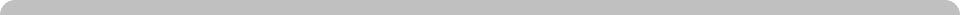Library of Psi Articles and Classes
Information
Database Group:
Magick

Path in Psi:
Magick

Content Type:
Article

Posted On:
Not Listed

Edited On:
2007-06-24

Letter Values
by Fire_Opal

Many numerological systems in use today still employ the Greek alphabet, so that the letter G is given the value of (3). It should be clear that the third letter of the English alphabet is C, not G. Using the English alphabet we find the following correspondence between numbers and letters: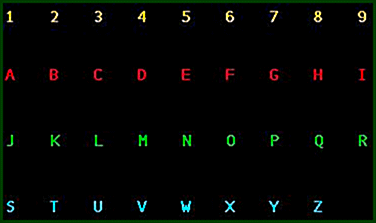In this way the letters A, J and S have the numerical value of (1), the letters B, K and T have the numerical value of 2, and so on. We can now analyze words and names and find out what they mean symbolically according to what they consist of numerologically. Firstly: the name is turned into a sequence of numbers.
Here is a sample name that can be translated into the number sequence:

This number sequence already gives a numerologist the basis for interpreting the name, say through character analysis of the name number.

Name Numbers

Most modern systems go no further than this name number. It is found by making a sum of the digits for the name to be interpreted. In the example given here the name number is (7).

Norman = 5 + 6 + 9 + 4 + 1 + 5 = 30 = 3 + 0 = (3)
Mortimer = 4 + 6 + 9 + 2 + 9 + 4 + 5 + 9 = 48 = 4 + 8 = 12 = 1 + 2 = (3)
Shine = 1 + 8 + 9 +5 + 5 = 28 = 2 + 8 = 10 = 1 + 0 = (1)

Norman Mortimer Shine = 3 + 3 + 1 = (7)

According to the interpretation of the symbolism of numbers we can give an analysis based on this name number.
This is, however a very superficial way of looking at numerology - and at names. Most people know their astrological sign of the zodiac, but anyone who has ever had a horoscope drawn up for the moment of birth has discovered that the day of birth only gives one factor and that being, say, an Aries is something that is shared with approximately one-twelth of the human race. There is a vast amount of extra data to be found in a personal horoscope. In the same way name numbers say much, but not nearly enough.
Please take the time to go thru the rest of the numerology section to learn more in-depth information.